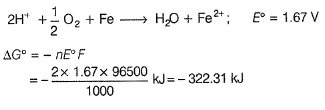Courses

# Retro (Past 13 Year) IIT-JEE Advanced (Electrochemistry)

## 18 Questions MCQ Test Chemistry for JEE Advanced | Retro (Past 13 Year) IIT-JEE Advanced (Electrochemistry)

Description
This mock test of Retro (Past 13 Year) IIT-JEE Advanced (Electrochemistry) for JEE helps you for every JEE entrance exam. This contains 18 Multiple Choice Questions for JEE Retro (Past 13 Year) IIT-JEE Advanced (Electrochemistry) (mcq) to study with solutions a complete question bank. The solved questions answers in this Retro (Past 13 Year) IIT-JEE Advanced (Electrochemistry) quiz give you a good mix of easy questions and tough questions. JEE students definitely take this Retro (Past 13 Year) IIT-JEE Advanced (Electrochemistry) exercise for a better result in the exam. You can find other Retro (Past 13 Year) IIT-JEE Advanced (Electrochemistry) extra questions, long questions & short questions for JEE on EduRev as well by searching above.
QUESTION: 1

### In a galvanic cell, the salt bridge

Solution:

This problem is based on the characteristics of salt-bridge.
Functions of salt-bridge

(i) It connects the two half-cells and completes the cell circuit.

(ii) It keeps the solutions of two half-cells and complete the cell circuit but does not participate chemically in the cell reaction.

(iii) It maintains the diffusion of ions from one electrode to another electrode.

(iv) A cell reaction may also occur in the absence of salt-bridge. Sometimes, both the electrodes dip in the same electrolyte solution and in such cases we do not require a salt-bridge.” So, option (c) is incorrect.

(v) This prevent mixing of two electrolytic solutions hence, option (d) is incorrect.

QUESTION: 2

### An aqueous solution of X is added slowly to an aqueous solution of Y as shown in Column I. The variation in conductivity of these reactions is given in Column II. Match Column I with Column II and select the correct answer using the codes given below.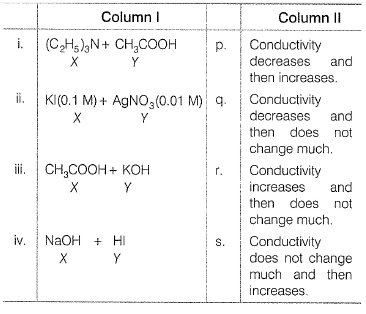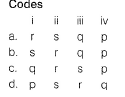Solution:

(i) X and Y both are weak electrolytes, hence have minimum conductance. When X [(C2H5)3N] is added to Y(CH3COOH), ions are formed hence conductance increases.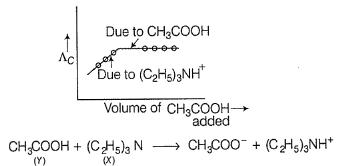After complete reaction further addition of Y,makes no change in conductance.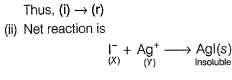where X (I-) is added to Y (Ag+) insoluble Agl is formed, Hence, there is no change in conductance. After complete reaction of Ag+, further addition of I- causes increase in conductance.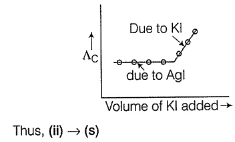(iii) KOH is a strong electrolyte. As CH3COOH(X)is added, OH- is neutralised by CH3COOH.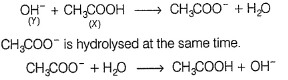But being in small extent, conductance decreases. After complete reaction of KOH, further addition of CH3COOH, (weak) there is no change in conductance.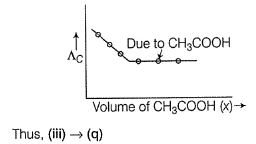(iv) NaOH(X) and HI(Y) both are strong electrolytes. Thus, net reaction is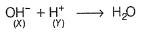When (X) is added to (V), H2O is formed hence conductance decreases. After complete reaction of OH-, further addition of NaOH (X), causes increases in conductance.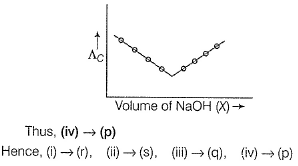QUESTION: 3

### The standard reduction potential data at 25°C is given below.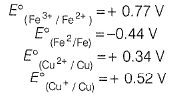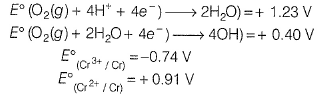Match E° of the rebox pair in Column I with the values given in Column II and select the correct answer using the codes given below.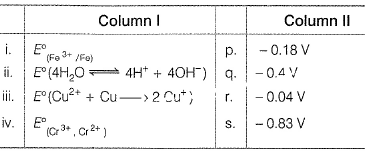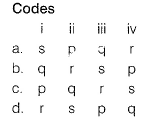(2013 Adv., Matching Type)

Solution:

Plan When different number of electrons are involved in a redox reaction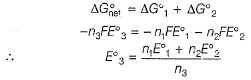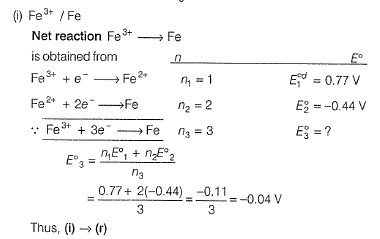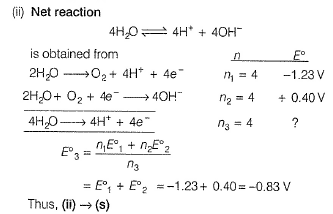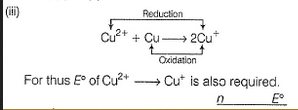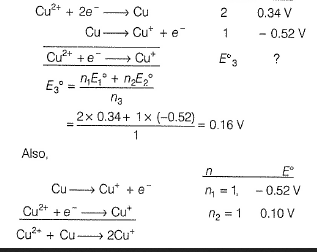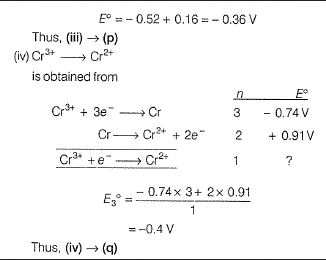QUESTION: 4

Passage for Q. Nos. (4-5)

The electrochemical cell shown below is a concentration cell. M | M2+ (saturated solution of a sparingly soluble salt, MX2) | | M2+ (0.001 mol dm-3) |M The emf of the cell depends on the difference in concentration of M2+ ions at the two electrodes. The emf of the cell at 298K is 0.059 V.

Q.

The solubility product (Ksp ; mol3dm-9) of MX2 at 298 K based on the information available the given concentration cell is (take 2.303x R x 298 / F= 0.059 V)

Solution: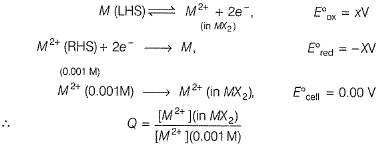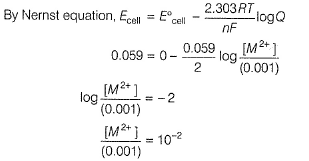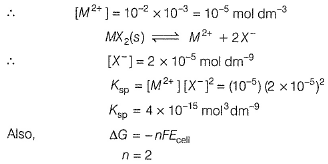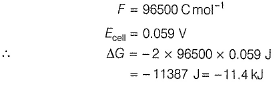QUESTION: 5

The electrochemical cell shown below is a concentration cell. M | M2+ (saturated solution of a sparingly soluble salt, MX2) | | M2+ (0.001 mol dm-3) |M The emf of the cell depends on the difference in concentration of M2+ ions at the two electrodes. The emf of the cell at 298K is 0.059 V.

Q.

The value of ΔG (kJ mol-1) for the given cell is (take 1 F= 96500 C mol-1)

Solution:QUESTION: 6

Consider the following cell reaction,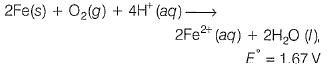At [Fe2+] = 10-3 M, p(O2) = 0.1 atm and pH = 3, the cell potential at 25°C is

(2011, Only One Option Correct Type)

Solution:

The half reactions are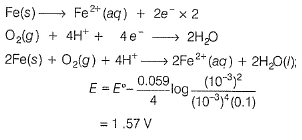QUESTION: 7

AgNO3 (aqueous) was added to an aqueous KCI solution gradually and the conductivity of the solution was measured. The plot of conductance (A) versus the volume of AgNO3 is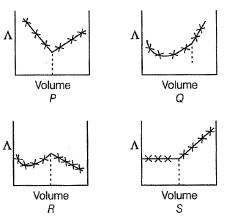(2011, Only One Option Correct Type)

Solution:

As AgNO3 is added to solution , KCI will be displaced according to the following reaction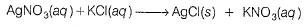For every mole of KCI displaced from solution, one mole of KNO3 comes in solution resulting in almost constant conductivity. As the end point is reached, added AgNO3 remain in solution increasing ionic concentration, hence conductivity increases.

QUESTION: 8

Passage for Q. N os. (8-9)

The concentration of potassium ions inside a biological cell is at least twenty times higher than the outside. The resulting potential difference across the cell is important in several processes such as transmission of nerve impulses and maintaining the ion balance. A simple model for such a concentration cell involving a metal M is M(s) | M+ (aq 0.05 molar) | | M+ (ag; 1 molar) | M(s).
For the above electrolytic cell the magnitude of the cell potential | Ecell  | = 70 mV.

Q.

For the above cell

Solution: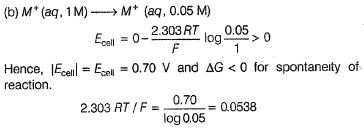QUESTION: 9

The concentration of potassium ions inside a biological cell is at least twenty times higher than the outside. The resulting potential difference across the cell is important in several processes such as transmission of nerve impulses and maintaining the ion balance. A simple model for such a concentration cell involving a metal M is M(s) | M+ (aq 0.05 molar) | | M+ (ag; 1 molar) | M(s).
For the above electrolytic cell the magnitude of the cell potential | Ecell  | = 70 mV.

Q.

If the 0.05 molar solution of M+ is replaced by a 0.0025 molar M+ solution, then the magnitude of the cell potential would be

Solution: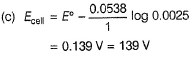*Multiple options can be correct
QUESTION: 10

For the reduction of NO3- ion in an aqueous solution E° is + 0.96 V. Values of E° for some metal ions are given below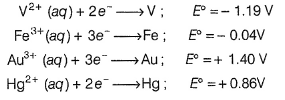The pair(s) of metals that is(are) oxidised by NO3- in aqueous solution is(are)

(2009, One or More than One Options Correct Type)

Solution:

Metals with E° value less than 0.96 V will be able to reduce NO3- in aqueous solution.Therefore, metals V (E° = - 1.19 V), Fe (E° = - 0.04 V), Hg (E° = 0.86 'V) will all reduce NO3- but Au (E° = 1.40 V) cannot reduce NO3- in aqueous solution.

QUESTION: 11

Electrolysis of dilute aqueous NaCI solution was carried out by passing 10 mA current. The time required to liberate 0.01 mole of H2 gas at the cathode is (1 F = 96500 C mol-1)

(2008,Only One Option Correct Type)

Solution:

0.01 mol H2 = 0.02 g equivalent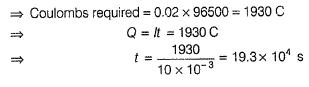QUESTION: 12

Passage for Q. Nos. (12-13)

Redox reaction play a vital role in chemistry and biology. The values of standard redox potential (E°) of two half-cell reactions decide which way the reaction is expected to proceed. A simple example is a Daniell cell in which zinc goes into solution and copper gets deposited. Given below are a set of half-cell reactions (acidic medium) along with their E° (V with respect to normal hydrogen electrode) values.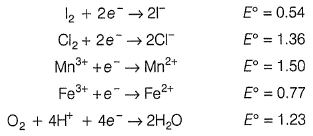Q.

Among the following, identify the correct statement.

Solution:

For spontaneous redox reaction : E°cell > 0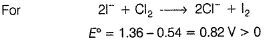i.e. Cl2 will spontaneously oxidise l- .
In other cases E°cell < 0, they are non-spontaneous.

QUESTION: 13

Redox reaction play a vital role in chemistry and biology. The values of standard redox potential (E°) of two half-cell reactions decide which way the reaction is expected to proceed. A simple example is a Daniell cell in which zinc goes into solution and copper gets deposited. Given below are a set of half-cell reactions (acidic medium) along with their E° (V with respect to normal hydrogen electrode) values.Q.

While Fe3+ is stable, Mn3+ is not stable in acid solution because

Solution:

For the reaction,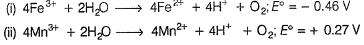As evidenced above, reaction (i) is non-spontaneous, therefore, Fe3+ is stable in acid solution. However, reaction (ii) is spontaneous Me3+ oxidises H2O to O2 and itself reduced to Mn2+ in acidic medium.

QUESTION: 14

Passage for Q. Nos. (14-16)

Tollen’s reagent is used for the detection of aldehydes. When a solution of AgNO3 is added to glucose with NH4OH, then gluconic acid is formed.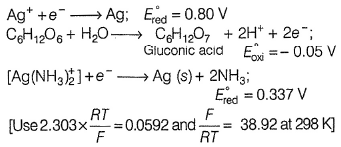Q.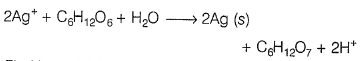Find In K of this reaction

Solution: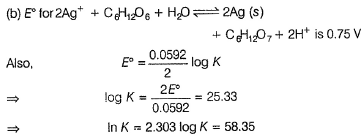QUESTION: 15

Tollen’s reagent is used for the detection of aldehydes. When a solution of AgNO3 is added to glucose with NH4OH, then gluconic acid is formed.Q.

When ammonia is added to the solution, pH is raised to 11. Which half-cell reaction is affected by pH and by how much?

Solution:

On increasing concentration of NH3, the concentration of H+ ion decreases, therefore,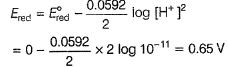i.e. Ered increases by 0.65 V.

QUESTION: 16

Tollen’s reagent is used for the detection of aldehydes. When a solution of AgNO3 is added to glucose with NH4OH, then gluconic acid is formed.Q.

Ammonia is always added in this reaction. Which of the following must be incorrect?

Solution:

NH3 has no effect on the E° of glucose/gluconic acid electrode.

*Answer can only contain numeric values
QUESTION: 17

We have taken a saturated solution of AgBr, Ksp is 12 x 10-14 If 10-7 M of AgNO3 are added to 1 L of this solution, find conductivity (specific conductance) of this solution in terms of 10-7 Sm-1 units.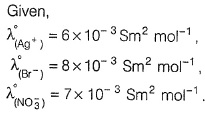(2006, Subjective Type)

Solution:

The solubility of AgBr in 10-7 M AgNO3 solution is determined as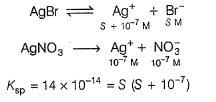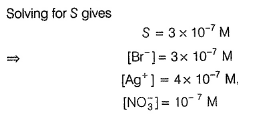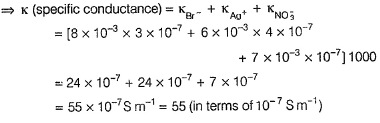QUESTION: 18

The half-cell reactions for rusting of iron are: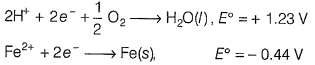ΔG° (in kJ) for the reaction is

(2005, Only One Option Correct Type)

Solution:

The net reaction is